# 30 Facts About Numbers That’ll Make You Feel Like a Mathematical Genius

## 21Longest mathematical proof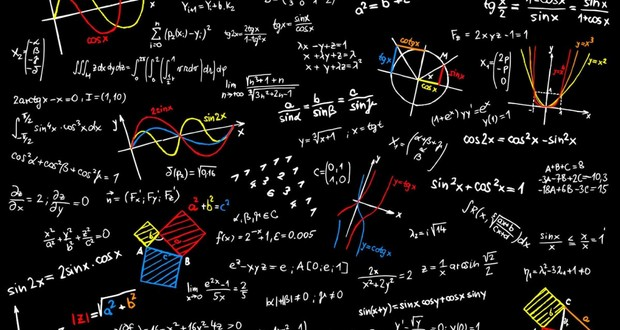The longest mathematical proof is 15,000 pages long, involved more than 100 mathematicians and took 30 years just to complete it.

## 22Per Enflo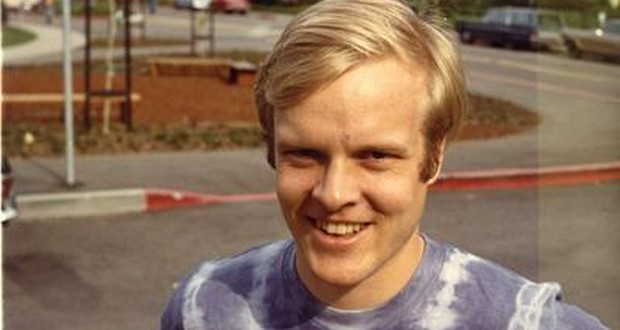A mathematician named Per Enflo solved Problem 153 in the "Scottish Book" in 1972 and was awarded a live goose as promised by Polish mathematician named Stanisław Mazur, who posed the problem in 1936.

## 23Vi HartIn 2012, a mathematician named Vi Hart sent an open letter to Nickelodeon proving Spongebob's Pineapple was geometrically impossible. Pineapple designer Kenny Pittenger responded on his blog (in video description) by posting a new "mathematically correct" design. This new design is now used on the show.

## 248675309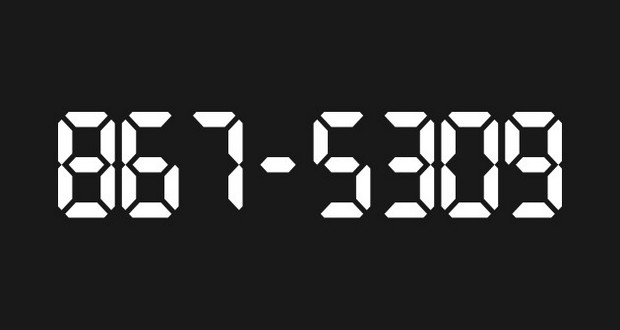8675309 is the fourth most common 7-digit password, number one, of course, is 1234567.

## 25Narcissistic numberA Narcissistic number is a number that is the sum of its own digits each raised to the power of the number of digits.

## 26VinculumThe line between the 2 numbers in a fraction is called the Vinculum.

## 27Fry Lewis RichardsonA mathematician named Fry Lewis Richardson discovered that it is impossible to measure the length of coastlines in a meaningful manner. This so-called Coastline Paradox later led to the creation of the field of Fractal Geometry.

## Latest FactRepublic Video: 32 Incredible Easter Eggs You Missed in Harry Potter MoviesA female English mathematician named Ada Lovelace wrote an algorithm in the mid-1800s designed to be carried out by a machine. As a result, she is often regarded as the first computer programmer.

## 29Integral symbol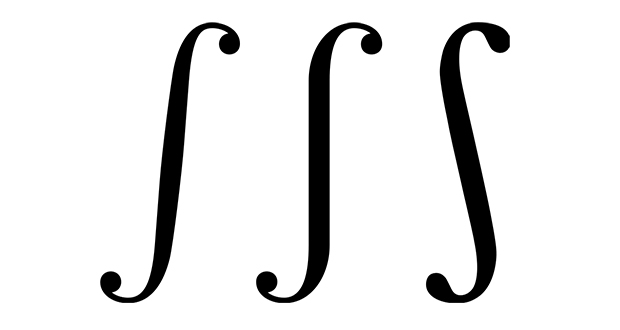The integral symbol (∫) used in math is based on the long S symbol because Leibniz thought of the integral as an infinite sum of infinitesimal summands.

## 30Johann Gustav Hermes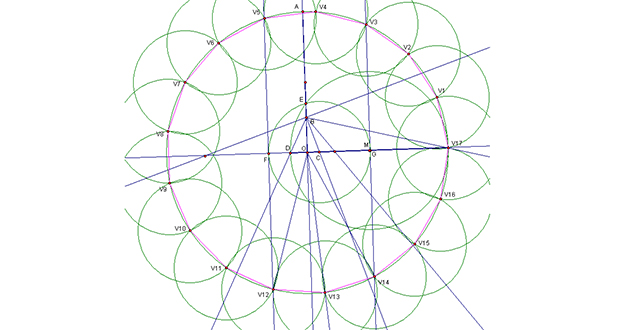A German mathematician named Johann Gustav Hermes spent a decade writing a 200-page manuscript about drawing a regular 65537 sided polygon with a compass and a straightedge.